• +91 9971497814
• info@interviewmaterial.com

# RD Chapter 23- The Straight Lines Ex-23.18 Interview Questions Answers

### Related Subjects

Question 1 :

Find the equation of the straight lines passing through the origin andmaking an angle of 45o with the straight line √3x + y = 11.

Given:

Equation passesthrough (0, 0) and make an angle of 45° with the line √3x + y = 11.

We know that, theequations of two lines passing through a point x1,y1 and making anangle α with the given line y = mx + c are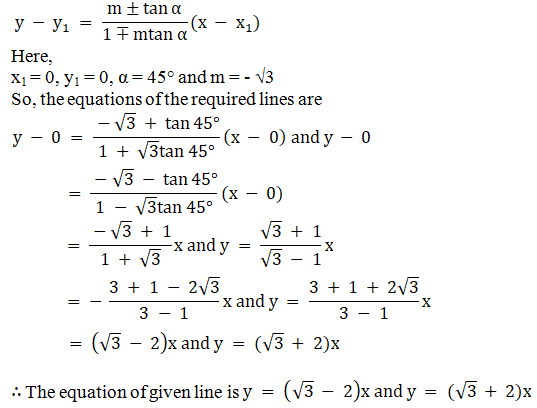Question 2 :

Find the equations to the straight lines which pass through the originand are inclined at an angle of 75o to the straight line x + y+ √3(y – x) = a.

Given:

The equation passesthrough (0,0) and make an angle of 75° with the line x + y + √3(y – x) = a.

We know that theequations of two lines passing through a point x1,y1 and making anangle α with the given line y = mx + c are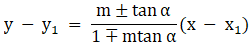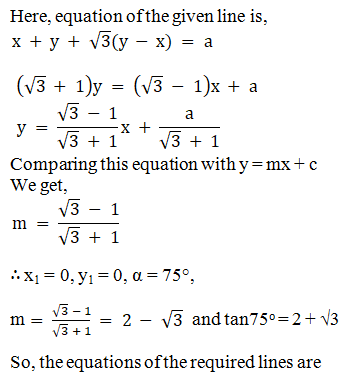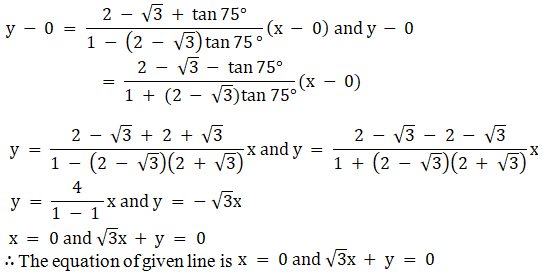Question 3 : Find the equations of straight lines passing through (2, -1) and making an angle of 45o with the line 6x + 5y – 8 = 0.

Given:

The equation passesthrough (2,-1) and make an angle of 45° with the line 6x + 5y – 8 = 0

We know that theequations of two lines passing through a point x1, y1 andmaking an angle α with the given line y = mx + c areHere, equation of thegiven line is,

6x + 5y – 8 = 0

5y = – 6x + 8

y = -6x/5 + 8/5

Comparing thisequation with y = mx + c

We get, m = -6/5

Where, x1 =2, y1 = – 1, α = 45°, m = -6/5

So, the equations ofthe required lines are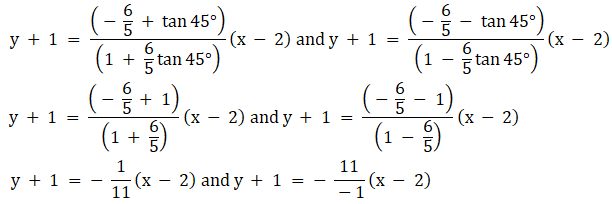x + 11y + 9 = 0 and11x – y – 23 = 0

The equation of givenline is x + 11y + 9 = 0 and 11x – y – 23 = 0

Question 4 : Find the equations to the straight lines which pass through the point (h, k) and are inclined at angle tan-1 m to the straight line y = mx + c.

Given:

The equation passesthrough (h, k) and make an angle of tan-1 m with theline y = mx + c

We know that theequations of two lines passing through a point x1, y1 andmaking an angle α with the given line y = mx + c are

m′ = m

So,Here,

x1 =h, y1 = k, α = tan-1 m, m′ = m.

So, the equations ofthe required lines are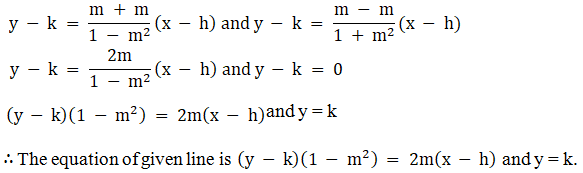Question 5 : Find the equations to the straight lines passing through the point (2, 3) and inclined at an angle of 450 to the lines 3x + y – 5 = 0.

Given:

The equation passesthrough (2, 3) and make an angle of 450with the line 3x + y – 5= 0.

We know that theequations of two lines passing through a point x1,y1 and making anangle α with the given line y = mx + c areHere,

Equation of the givenline is,

3x + y – 5 = 0

y = – 3x + 5

Comparing thisequation with y = mx + c we get, m = – 3

x1 =2, y1 = 3, α = 45, m = – 3.

So, the equations ofthe required lines are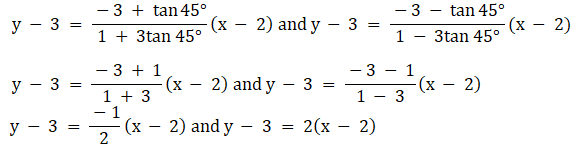x + 2y – 8 = 0 and 2x– y – 1 = 0

The equation of givenline is x + 2y – 8 = 0 and 2x – y – 1 = 0

Todays Deals### RD Chapter 23- The Straight Lines Ex-23.18 Contributorskrishan

Name:
Email:

# Latest News# 9000 interview questions in different categories